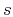Asymptotic pseudounitary stacking operatorsNext: Offset Continuation and DMO Up: EXAMPLES Previous: Post-Stack Time Migration

## Velocity Transform

Velocity transform is another form of hyperbolic stacking with the summation path(69)

wherecorresponds to the offset,is the stacking slowness, andis the estimated zero-offset traveltime. Hyperbolic stacking is routinely applied for scanning velocity analysis in common-midpoint stacking. Velocity transform inversion has proved to be a powerful tool for data interpolation and amplitude-preserving multiple suppression (Lumley et al., 1995; Thorson, 1984; Ji, 1995).

Solving equation (69) for, we find that the asymptotic inverse and adjoint operators have the elliptic summation path(70)

The weighting functions of the asymptotic pseudo-unitary velocity transform are found using formulas (28) and (29) to have the form(71)(72)

The factorfor pseudo-unitary velocity transform weighting has been discovered empirically by Claerbout (1995).

Figure 4 shows the output of a numerical test of the least-squares velocity transform inversion using a CMP gather from the Mobil AVO dataset (Lumley et al., 1995). The input CMP gather (shown in the left plot of Figure 5) is inverted using an iterative conjugate-gradient method and two different weighting scheme: the uniform weighting and the asymptotic pseudo-unitary weights (71-72). Analogously to Figure 1, the iterative convergence is measured by the least-squares norm of the data residual error at different iterations. Figure 4 shows that the pseudo-unitary weighting provides a noticeably faster convergence at the first three iterations. At later iterations, the residual errors of the two methods are very close to each other. The use of a pseudo-unitary weighting will be justified in this case if only three iterations are practically affordable. The results of inversion after 10 conjugate-gradient iterations are plotted in Figures 5 and 6. The right plot in Figure 5 shows the output of the velocity transform inversion: an optimized velocity scan. Figure 6 shows the corresponding modeled CMP gather and the residual error. The error is negligible which indicates a successful inversion.

cgiter
Figure 4.
Comparison of convergence of the iterative velocity transform inversion. The dashed line corresponds to the unweighted (uniformly weighted) operator. The solid line corresponds to the asymptotic pseudo-unitary operator. The latter provides a faster convergence at early iterations.dircvv
Figure 5.
Input CMP gather (left) and its velocity transform counterpart (right) after 10 iterations of iterative least-squares inversion.dirrst
Figure 6.
The modeled CMP gather (left) and the residual error (right) plotted at the same scale.Asymptotic pseudounitary stacking operatorsNext: Offset Continuation and DMO Up: EXAMPLES Previous: Post-Stack Time Migration

2013-03-03